# Line segment Problems

#### Number of problems found: 60

• Midpoint of segmentPoint A has coordinates [-16; 23] and the midpoint of the segment AB is the point [2; 12]. What are the coordinates of point B?
• Slope formFind the equation of a line given the point X(8, 1) and slope -2.8. Arrange your answer in the form y = ax + b, where a, b are the constants.
• Line segmentFor the line segment whose endpoints are L[-1, 13] and M[18, 2], find the x and y value for the point located 4 over 7 the distance from L to M.
• Slope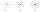Calculate the slope of a line that intersects points (-84,41) and (-76,-32).
• Segment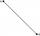Calculate the length of the segment AB, if the coordinates of the end vertices are A[10, -4] and B[5, 5].
• Perpendicular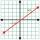What is the slope of the perpendicular bisector of line segment AB if A[9,9] and B[9,-2]?
• SegmentsWhich of the pairs of numbers on the number line encloses the longest segment: ?
• Cone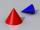If the segment of the line y = -3x +4 that lies in quadrant I is rotated about the y-axis, a cone is formed. What is the volume of the cone?
• Distance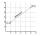Calculate distance between two points X[18; 19] and W[20; 3].
• Center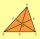In the triangle ABC is point D[1,-2,6], which is the center of the |BC| and point G[8,1,-3], which is the center of gravity of the triangle. Find the coordinates of the vertex A[x,y,z].
• LineCan we construct a line segment, if we know: center
• Right triangle from axesA line segment has its ends on the coordinate axes and forms with them a triangle of area equal to 36 square units. The segment passes through the point ( 5,2). What is the slope of the line segment?
• Construction of trapezoidConstruct a trapezoid if b = 4cm, c = 7cm, d = 4,5cm, v = 3 cm (Procedure, discussion, sketch, analysis, construction)
• CoordinatesDetermine the coordinates of the vertices and the content of the parallelogram, the two sides of which lie on the lines 8x + 3y + 1 = 0, 2x + y-1 = 0 and the diagonal on the line 3x + 2y + 3 = 0
• Three points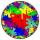Mark three points E, F and G in the plane not lie on one line. a) Draw a line segment FG b) Construct halfline (ray) EG c) Draw a line EF
• Draw a trapezoidDraw a trapezoid if given a = 7 cm, b = 4 cm, c = 3.5 cm, diagonal AC = 5cm. Solve as a construction task.
• Place vectorPlace the vector AB, if A (3, -1), B (5,3) in the point C (1,3) so that AB = CO
• Line segment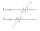Line segment AB is 8 cm long. Divide it in a ratio of 2: 3.
• Square ABCDConstruct a square ABCD with cente S [3,2] and the side a = 4 cm. Point A lies on the x-axis. Construct square image in the displacement given by oriented segment SS'; S` [-1 - 4].
• Medians and sides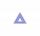Determine the size of a triangle KLM and the size of the medians in the triangle. K=(-5; -6), L=(7; -2), M=(5; 6).

Do you have an interesting mathematical word problem that you can't solve it? Submit a math problem, and we can try to solve it.

We will send a solution to your e-mail address. Solved examples are also published here. Please enter the e-mail correctly and check whether you don't have a full mailbox.

Please do not submit problems from current active competitions such as Mathematical Olympiad, correspondence seminars etc...